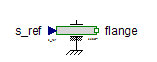Multiphase Power Sensor - MapleSim Help

Multiphase Power Sensor

Sense multiphase electrical powerDescription The Multiphase Power Sensor (or Power Sensor) component has $m$ current sensors and $m$ voltage sensors. The corresponding current and voltage  outputs are multiplied and the sum of products is output as the power.Equations $P=\sum _{k=1}^{m}{i}_{k}{v}_{k}$ ${v}_{k}={v}_{{p}_{k}}-{v}_{{n}_{k}}$ ${i}_{k}={i}_{{p}_{k}}=-{i}_{{n}_{k}}$Connections

 Name Description Modelica ID $\mathrm{pc}$ Positive plug, current path pc $\mathrm{nc}$ Negative plug, current path nc $\mathrm{pv}$ Positive plug, voltage path pv $\mathrm{nv}$ Negative plug, voltage path nv $\mathrm{power}$ Real output signal; power in $W$ powerParameters

 Name Default Units Description Modelica ID $m$ $3$ Number of phases mModelica Standard Library The component described in this topic is from the Modelica Standard Library. To view the original documentation, which includes author and copyright information, click here.